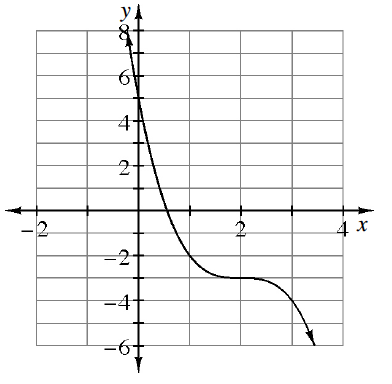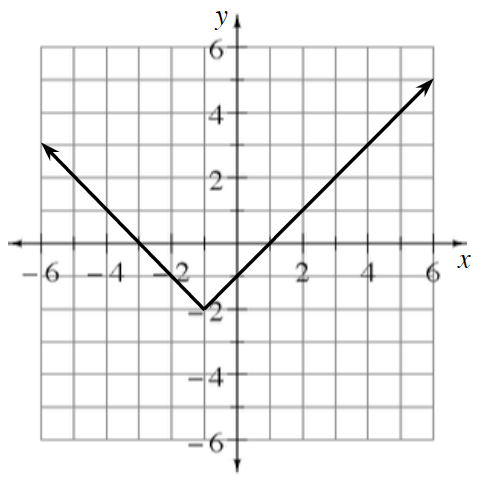### Home > PC3 > Chapter 2 > Lesson 2.2.5 > Problem2-114

2-114.

Each graph below is a transformation of one of the parent graphs. Write an equation for each graph below.

1.$\textit{y} = \textit{x}^3$

1. Reflect across $y$-axis → $y=−x^3$
2. Shifted right $2$ units → $y=−(x−2)^3$
3. Shifted down $3$ units → $y=−(x−2)^3−3$

1.$y = |x|$

$\text{Shifted left 1 unit}\Rightarrow y =\left | x+1 \right |$

$\text{Shifted down 2 units}\Rightarrow y =\left | x+1 \right |-2$